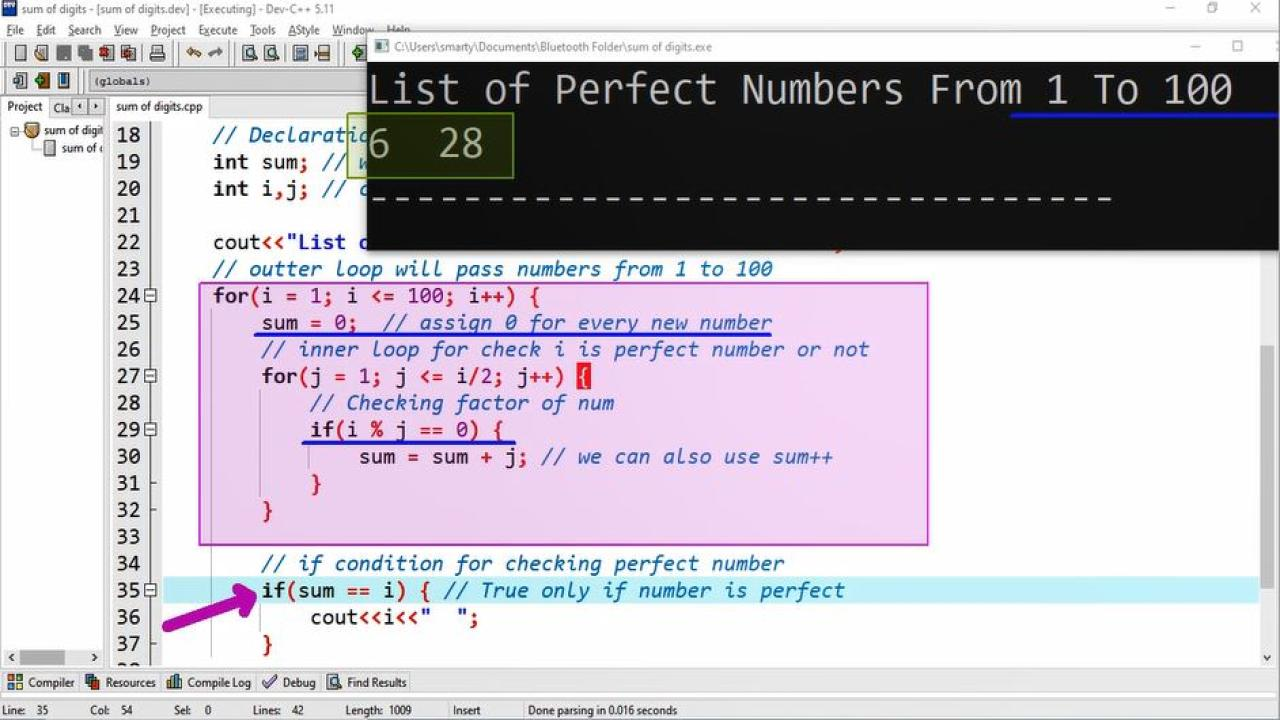# Perfect Numbers From 1 To 100 using CPP

## Source Code:

#include <iostream>

// standard namespace
using namespace std;

/*
A number N is called perfect number
iff the sum of its factors is equal
to the number.
For example: if N = 6
Factors of N are 1,2,3
and 1+2+3 = 6
So that 6 is perfect number
*/

int main(int argc, char** argv) {

// Declaration statements
int sum; // will store sum of factors.
int i,j; // counter variables.

// outter loop will pass numbers from 1 to 100
for(i = 1; i <= 100; i++) {
sum = 0;  // assign 0 for every new number
// inner loop for check i is perfect number or not
for(j = 1; j <= i/2; j++) {
// Checking factor of num
if(i % j == 0) {
sum = sum + j; // we can also use sum++
}
// end of inner loop

// if condition for checking perfect number
if(sum == i) { // True only if number is perfect
cout<<i<<"  ";
}
// end of outter loop

return 0;
}

## Output:## Working:

This program example use the code of already discussed program on instms So that for better understanding of how perfect numbers are calculated we can learn from Perfect number program using C++.

Additionally in this source code we also use an outter loop to check and print series of perfect number. In outter loop we start counter variable from 1 and goes upto 100. We can also change this 100 to our requirement. The ouuter loop pass value of counter variable j one after another to inner loop and inner loop check each value either it is perfect number or not. If the value of outter loop will fullfil the condition of perfect number then we will print it using cout statement, otherwise number will not print.

In start of outter loop every time we assign 0 to sum variable, so that it can hold sum of factors for next number.